Successfully reported this slideshow.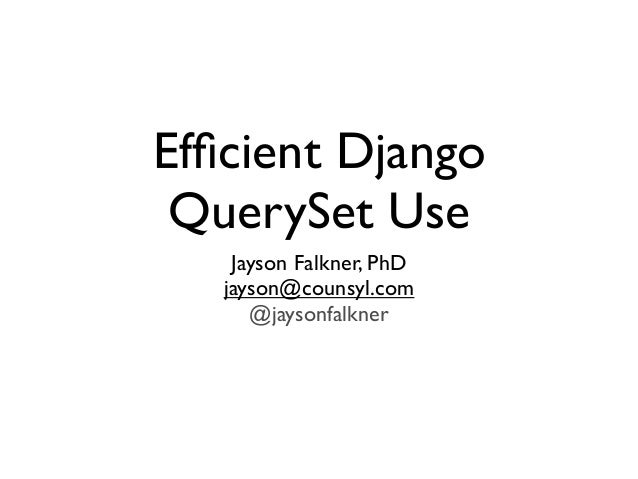Upcoming SlideShare
×

of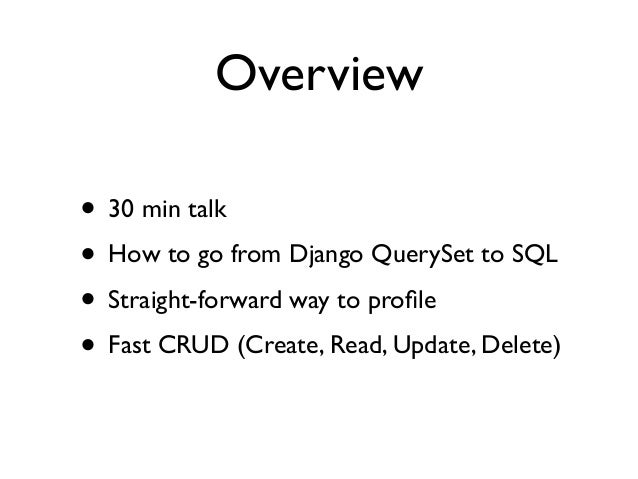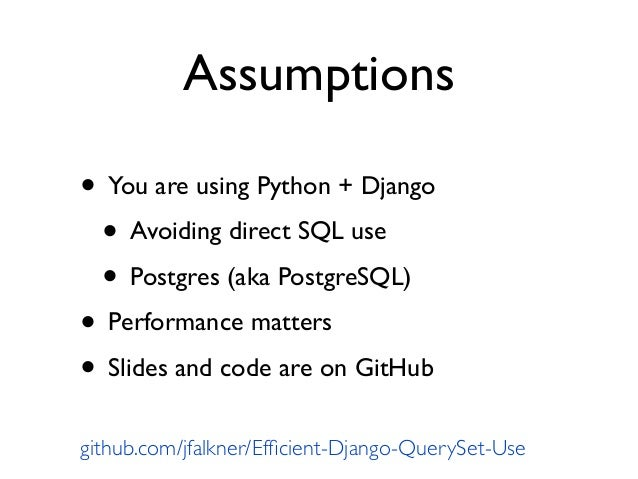Upcoming SlideShare
How I Learned to Stop Worrying about Big Data and Love the Data That Actually Counts - Counsyl Tech Talk
Next

70 Likes

Share

# Efficient Django QuerySet Use

This talk is about advanced use of Django's QuerySet API based on lessons learned at Counsyl. You'll learn how to debug, optimize and use the QuerySet API efficiently. The main examples focus on demystifying how QuerySet maps to SQL and how seemingly simple Python code, presumably fast O(1) queries, can result in unacceptably long runtime and often O(N) or worse queries. Solutions are provided for keeping your Python code simple and RDMS use performant.

Code: https://github.com/jfalkner/Efficient-Django-QuerySet-Use

See all

See all

### Efficient Django QuerySet Use

1. 1. Efﬁcient Django QuerySet Use Jayson Falkner, PhD jayson@counsyl.com @jaysonfalkner
2. 2. Overview • 30 min talk • How to go from Django QuerySet to SQL • Straight-forward way to proﬁle • Fast CRUD (Create, Read, Update, Delete)
3. 3. Assumptions • You are using Python + Django • Avoiding direct SQL use • Postgres (aka PostgreSQL) • Performance matters • Slides and code are on GitHub github.com/jfalkner/Efﬁcient-Django-QuerySet-Use
4. 4. A simple data model class Sample(models.Model): barcode = models.CharField(max_length=10, unique=True) production = models.BooleanField() created = models.DateTimeField() def status(self): return self.statuses.all() class SampleStatus(models.Model): sample = models.ForeignKey(Sample, related_name='statuses') status_code = models.PositiveSmallIntegerField() created = models.DateTimeField() RECEIVED = 1; LAB = 2; COMPLETE = 3
5. 5. Don’t do this # What samples are in the lab? samples = Sample.objects.filter( production=True, statuses__status_code=SampleStatus.LAB) # Loop through. It’s business time! for s in samples: do_something(s.barcode, s.status().created)
6. 6. What takes time? Perform SQL Serialize ResultsParse SQL Runtime (Django +Your Code) Parse ResultsSerialize SQL Python Postgres (RDMS) Socket
7. 7. Poor Man’s Django/QuerySet Proﬁling from datetime import datetime from django.db import connection as con from example.models import Sample start = datetime.now() query_count = len(con.queries) samples = Sample.objects.filter( production=True, statuses__status_code =SampleStatus.LAB) for s in samples: do_something(s.barcode, s.status().created) print “Time: %s”%(datetime.now()-start) print “Queries: %s”%(len(con.queries)-query_count) for query in connection.queries[query_count:]: print query
8. 8. from datetime import datetime from django.db import connection as con from example.models import Sample, SampleStatus start = datetime.now() query_count = len(con.queries) samples = Sample.objects.filter( production=True, statuses__status_code = SampleStatus.LAB) for s in samples: do_something(s.barcode, s.status().created) print “Time: %s”%(datetime.now()-start) print “Queries: %s”%(len(con.queries)-query_count) for query in connection.queries[query_count:]: print query Poor Man’s Django/QuerySet Proﬁling
9. 9. from datetime import datetime from django.db import connection as con from example.models import Sample, SampleStatus import django_pg_utils django_pg_utils.track_sql() samples = Sample.objects.filter( production=True, statuses__status_code = SampleStatus.LAB) for s in samples: do_something(s.barcode, s.status().created) django_pg_utils.print_sql() Poor Man’s Django/QuerySet Proﬁling github.com/jfalkner/Efﬁcient-Django-QuerySet-Use
10. 10. # Read all matching samples. samples = Sample.objects.filter( production=True, statuses__status_code=SampleStatus.LAB) # Loop with query for `created` value. for s in samples: do_something(s.barcode, s.status().created) SQL Read in QuerySet (1,000 samples) Python:1.112s, Postgres: 0.009s, Queries: 1,001 (And one more for each other sample...) SELECT "id", "sample_id", "status", "created" FROM "db_samplestatus" WHERE "sample_id" = 10 ORDER BY "created" DESC LIMIT 1 SELECT "id", "barcode", "production", "created" FROM "db_sample" WHERE "db_sample"."production" = TRUE
11. 11. Why is it slow? • O(n) queries when it could be O(1) • One query for all samples • One query per status of sample • Serializes/deserializes unused values • Sample: id, created • SampleStatus: id, status, sample_id
12. 12. samples = Sample.objects.filter( production=True, statuses__status_code=SampleStatus.LAB) # Cache all lookups once in Python dict. samples = samples.prefetch_related(‘statuses’) for s in samples: do_something(s.barcode, s.status().created) SQL Read in QuerySet (1,000 samples) Python: 0.460s, Postgres: 0.018s, Queries: 2 SELECT "id", "barcode", "production", "created" FROM "db_sample" INNER JOIN "db_samplestatus" ON ("id" = "db_samplestatus"."sample_id") WHERE ("production" = true AND "db_samplestatus"."status_code" = 2 ) SELECT "id", "sample_id", "status_code", "created" FROM "db_samplestatus" WHERE "sample_id" IN (1220001, 1220002, 1220003 ...
13. 13. Improving even more • O(1) query = Good • Python dict for lookups is relatively slow • Let Postgres do the work • Return only params of interest • Minimizes serialize/deserialize time • Smaller memory footprint
14. 14. Sample.objects # JOIN and calc max status code from SampleStatus. .annotate(latest_status_code=Max( 'statuses__status_code')) .filter(production=True, latest_status_code__eq=SampleStatus.LAB) # Return exactly the fields to use. .values_list('barcode', 'statuses__created') SQL Read in QuerySet (10,000 samples) Python: 0.23s, Postgres: 0.18s, Queries: 1 SELECT "barcode", "created" FROM "db_sample" LEFT OUTER JOIN "db_samplestatus" ON ("id" = "db_samplestatus"."sample_id") WHERE ("production" = TRUE) GROUP BY "id", "barcode", "production", "created", "status_code", "db_samplestatus"."status_code" HAVING MAX("db_samplestatus"."status_code") = 2
15. 15. class Sample(models.Model): barcode = models.CharField(max_length=10, unique=True) production = models.BooleanField() created = models.DateTimeField() latest_status = models.ForeignKey( 'SampleStatus', related_name='+', null=True) def status(self): return self.statuses.all() class SampleStatus(models.Model): ... Using select_related() JOIN via denormalized foreign key on Sample
16. 16. samples = Sample.objects.filter( production=True, statuses__status_code=SampleStatus.LAB) samples = samples.select_related('latest_status') for s in samples: do_something((s.barcode, s.latest_status.created)) SQL Read in QuerySet (10,000 samples) Python: 0.71s, Postgres: 0.13s, Queries: 1 SELECT "id", "barcode", "production", "created", "latest_status_id", T3."id", T3."sample_id", T3."status", T3."status_code", T3."created" FROM "example_sample" INNER JOIN "example_samplestatus" ON ("id" = "example_samplestatus"."sample_id") LEFT OUTER JOIN "example_samplestatus" T3 ON ("latest_status_id" = T3."id") WHERE ("production" = TRUE AND "example_samplestatus"."status_code" = 2)
17. 17. class Sample(models.Model): barcode = models.CharField(max_length=10, unique=True) production = models.BooleanField() created = models.DateTimeField() status_code = models.PositiveSmallIntegerField() status_changed = models.DateTimeField() def status(self): return self.statuses.all() class SampleStatus(models.Model): ... Denormalize for speed Change the data model to avoid a JOIN
18. 18. # Read all at once and return a list of tuples. Sample.objects .filter(production=True, status_code = SampleStatus.LAB) .values_list('barcode', 'status_changed') SQL Read in QuerySet (1,000,000 samples) Python: 0.18s, Postgres: 0.17s, Queries: 1 SELECT "db_sample"."barcode", "db_sample"."status_code" FROM "db_sample" WHERE ("db_sample"."status_code" = 2 AND "db_sample"."production" = TRUE)
19. 19. Why is it faster? • JOINs and table scans are relatively slow • SQL’s EXPLAIN helps show this postgresql.org/docs/9.1/static/using-explain.html EXPLAIN SELECT "db_sample"."barcode", "db_sample"."status_code" FROM "db_sample" WHERE ("db_sample"."status_code" = 2 AND "db_sample"."production" = TRUE) Seq Scan on db_sample (cost=0.00..27203.00 rows=5567 width=8) Filter: (production AND (status_code = 2))
20. 20. GroupAggregate (cost=691622.75..787997.34 rows=2965372 width=23) Filter: (max(db_samplestatus.status_code) = 2) -> Sort (cost=691622.75..699036.18 rows=2965372 width=23) Sort Key: id, barcode, production, created, status_code, -> Hash Right Join (cost=43063.00..190400.49 rows=2965372 width=23) Hash Cond: (db_samplestatus.sample_id = db_sample.id) -> Seq Scan on db_samplestatus (cost=0.00..51015.90 rows=2993590 width=6) -> Hash (cost=24703.00..24703.00 rows=1000000 width=21) -> Seq Scan on db_sample (cost=0.00..24703.00 rows=1000000 width=21) Filter: production SQL Read in QuerySet (1,000,000 samples) Denormalized = 0.175s Seq Scan on db_sample (cost=0.00..27203.00 rows=5567 width=8) Filter: (production AND (status_code = 2)) Sample, SampleStatus JOIN = 11s Denormalized + Multicolumn INDEX = 0.004s Index Scan using db_sample_prod_lab on db_sample (cost=0.00..9101.15 rows=5567 width=8) Index Cond: ((status_code = 2) AND (production = true)) Filter: production
21. 21. class Sample(models.Model): barcode = models.CharField(max_length=10, unique=True) production = models.BooleanField() created = models.DateTimeField() status_code = models.PositiveSmallIntegerField(index=True) status_changed = models.DateTimeField() Multi-Column Indexing from django_pg_utils import pg_multicolumn_index pg_multicolumn_index(Sample, ['production', 'status_code']) Django Model ‘index’ won’t help Postgres-speciﬁc CREATE INDEX helper method
22. 22. How long does it take? QuerySet SELECT # Samples Time (Postgres) Loop 1,000 1.11s (0.01s) Loop prefetch_selected() 1,000 0.46s (0.02s) Loop select_related() 10,000 0.71s (0.13s) values_list() + JOIN 10,000 0.23s (0.19s) Denorm + INDEX 100,000,000 0.99s (0.38s) On a MacBook Pro Retina. Postgres 9.1. No conﬁg tweaks.
23. 23. In context at Counsyl • How much is 100,000,000 samples? • query: samples to process this week? • Consider Counsyl’s main product • 4,000,000 US pregnancies per year • Screen 2x people (mother and father) • That is 12 years of samples!
24. 24. Oh yeah, other stuff! • Batching CRUD actions = speed • Avoid any O(n) loops on model objects • Bulk CREATE, UPDATE, DELETE exist • bulk_create(), update(), delete() • save() won’t work • Helper code for multi-value UPDATE docs.djangoproject.com/en/dev/topics/db/queries/
25. 25. SELECT "id", "barcode", "production", "created" FROM "db_sample" WHERE "production" = TRUE SELECT (1) AS "a" FROM "db_sample" WHERE "id" = 1 LIMIT 1 samples = Sample.objects.filter(production=True) # Loop, update, and invoke `save()` for each. for s in samples: s.barcode = 'PREFIX'+s.barcode s.save() Inefﬁcient UPDATE in QuerySet Queries: O(n) UPDATE "db_sample" SET "barcode" = 'PREFIX0', "production" = TRUE, "created" = '2013-09-29' WHERE "db_sample"."id" = 1 (And one more for each other sample...)
26. 26. # Loop and make samples one at a time. now = datetime.now() (Sample.objects.filter(production=True) .update(created=now)) Efﬁcient UPDATE in QuerySet UPDATE "db_sample" SET "created" = '2013-09-29 03:40:18.925695-05:00' WHERE "db_sample"."production" = TRUE Queries: O(1)
27. 27. No multi-value update? • Django’s update() is limited to one value • SQL has no such restriction
28. 28. SET barcode = input.update FROM (SELECT unnest(ARRAY[1, 2, 3, 4, 5, 6]), unnest(ARRAY[6, 6, 6, 6, 6, 6])) AS INPUT (filter, UPDATE) WHERE id = INPUT.filter; values = Sample.objects.values_list('id', 'barcode') # Loop and make samples one at a time. filter_vals = [id for id, _ in values] update_vals = ['PREFIX'+bar for _, bar in values] django_pg_utils.pg_bulk_update(Sample, 'id', 'barcode', filter_vals, update_vals) Efﬁcient UPDATE in QuerySet Queries: O(1)
29. 29. # Loop and make samples one at a time. for barcode in barcodes: Sample.objects.create(barcode=barcode, production=True, created=datetime.now()) Inefﬁcient CREATE in QuerySet Queries: O(n) INSERT INTO "example_sample" ("barcode", "production", "created") VALUES ('01234', TRUE, '2013-10-04 01:55:15.456163-05:00', 2, '2013-10-04 01:55:15.456173-05:00') RETURNING "example_sample"."id" (And one more for each other sample...)
30. 30. # Buffer all Sample instances with values in a list. samples = [] for barcode in barcodes: samples.append(Sample(barcode=barcode, production=True, created=datetime.now()) # Bulk create all samples at once. Sample.objects.bulk_create(samples) Efﬁcient CREATE in QuerySet Queries: O(1) INSERT INTO "example_sample" ("barcode", "production", "created") VALUES ('100000', TRUE, '2013-10-04 02:00:01.556393-05:00'), ('100001', TRUE, '2013-10-04 02:00:01.556483-05:00'), ('100002', TRUE, '2013-10-04 02:00:01.556526-05:00'), ...
31. 31. Summary • Batch/Bulk Everything FTW • READ: values() and values_list() •pg_multicolumn_index() • CREATE: bulk_create() • UPDATE: update() and pg_bulk_update() • DELETE: delete() github.com/jfalkner/Efﬁcient-Django-QuerySet-Use
32. 32. Efﬁcient Django QuerySet Use Jayson Falkner, PhD jayson@counsyl.com @jaysonfalkner github.com/jfalkner/Efﬁcient-Django-QuerySet-Use
•#### AlexKo16

Jul. 2, 2020
•#### ciberglo

Aug. 23, 2016
•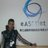#### mattnux

Jul. 13, 2016
•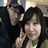#### kucuny

Jun. 27, 2016
•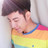#### allen__

Apr. 11, 2016
•#### ThiagoJourdan1

Apr. 7, 2016
•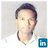#### HarishOraon

Mar. 16, 2016
•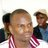#### nknganda

Mar. 2, 2016
•#### weihuiqiu

Feb. 1, 2016
•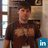#### EvgeniyDorogoy

Nov. 13, 2015
•#### bbirdd

Nov. 10, 2015
•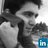Oct. 1, 2015
•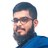#### AmrullahZunzunia

Sep. 29, 2015
•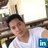#### JonardRosas

Aug. 30, 2015
•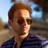#### chintamanil

Aug. 20, 2015
•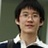#### nattster

Aug. 17, 2015
•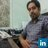Jul. 4, 2015
•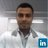#### FelipePalma5

May. 24, 2015
•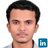#### ajeebkp23

Apr. 22, 2015
•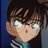#### jayuloy

Mar. 16, 2015

Total views

25,457

On Slideshare

0

From embeds

0

Number of embeds

4,927

251

Shares

0